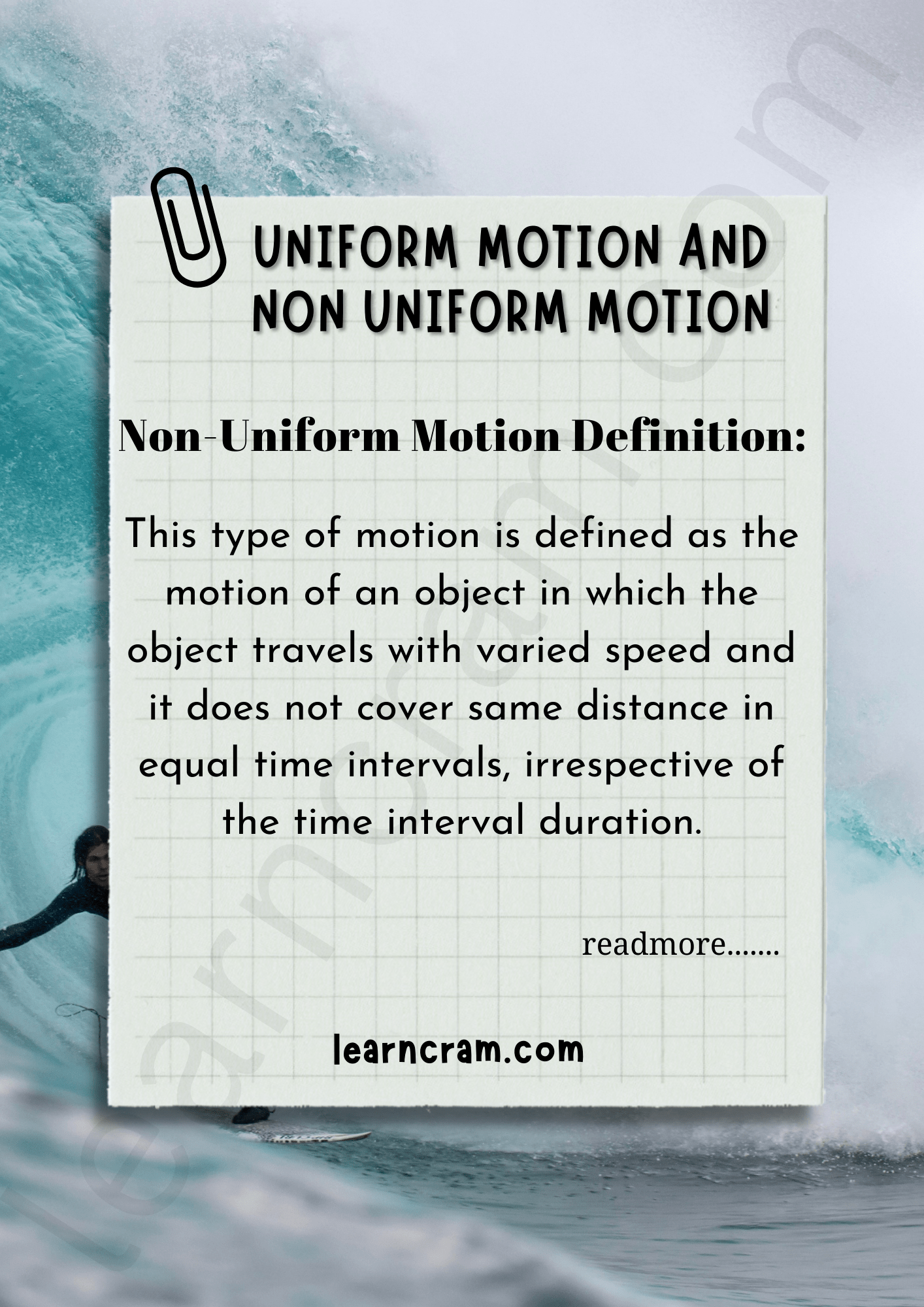# Uniform Motion and Non Uniform Motion | Definition, Examples – Motion in a Straight Line

## Uniform Motion and Non- Uniform Motion | Definition, Examples – Motion in a Straight Line

We are giving a detailed and clear sheet on all Physics Notes that are very useful to understand the Basic Physics Concepts.

Uniform Motion Definition:
If an object is moving along the straight line covers equal distance in equal interval of time, it is said to be in uniform motion along a straight line.Uniform Motion Example:

• A car moves at a constant speed on a straight path. At certain points travel time and traveled distance are measured and recorded.
• A vibrating spring in a sewing machine.

Non-Uniform Motion Definition:
This type of motion is defined as the motion of an object in which the object travels with varied speed and it does not cover same distance in equal time intervals, irrespective of the time interval duration.Non-Uniform Motion Example:

• Movement of an asteroid
• Dragging a box from a path

Motion in a Straight Line Topics: